Next: Bibliography Up: The Octonions Previous: Brougham Bridge

On Quaternions and Octonions: Their Geometry, Arithmetic, and Symmetry
by John H. Conway and Derek A. Smith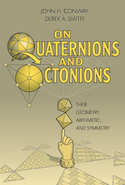review by John C. Baez

August 12, 2004

Published in Bull. Amer. Math. Soc. 42 (2005), 229-243.

Also available in Postscript and PDF formats

Conway and Smith's book is a wonderful introduction to the normed division algebras: the real numbers ($$\mathbb{R}$$), the complex numbers ($$\mathbb{C}$$), the quaternions ($$\mathbb{H}$$) and the octonions ($$\mathbb{O}$$). The first two are well-known to every mathematician. In constrast, the quaternions and especially the octonions are sadly neglected, so the authors rightly concentrate on these. They develop these number systems from scratch, explore their connections to geometry, and even study number theory in quaternionic and octonionic versions of the integers.

Conway and Smith warm up by studying two famous subrings of $$\mathbb{C}$$: the Gaussian integers and Eisenstein integers. The Gaussian integers are the complex numbers $$x + iy$$ for which $$x$$ and $$y$$ are integers. They form a square lattice: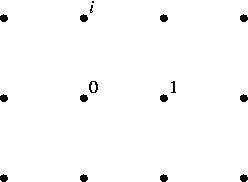Any Gaussian integer can be uniquely factored into 'prime' Gaussian integers — at least if we count differently ordered factorizations as the same, and ignore the ambiguity introduced by the invertible Gaussian integers, namely $$\pm 1$$ and $$\pm i$$. To show this, we can use a straightforward generalization of Euclid's proof for ordinary integers. The key step is to show that given nonzero Gaussian integers $$a$$ and $$b$$, we can always write $a = nb + r$ for some Gaussian integers $$n$$ and $$r$$, where the 'remainder' $$r$$ has $|r| < |b| .$ This is equivalent to showing that we can find a Gaussian integer $$n$$ with $|a/b - n| < 1 .$ And this is true, because no point in the complex plane has distance $$\ge 1$$ to the nearest Gaussian integer.

Similarly, the Eisenstein integers are complex numbers of the form $$x + \omega y$$ where $$x$$ and $$y$$ are integers and $$\omega$$ is a nontrivial cube root of $$1$$. These form a lattice with hexagonal symmetry: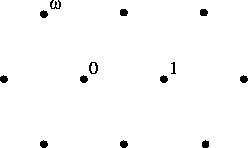Again one can prove unique factorization up to reordering and units, using the fact that no point in the complex plane has distance $$\ge 1$$ to the nearest Eisenstein integer.

To see the importance of this condition, consider the 'Kummer integers': numbers of the form $$x + \sqrt{-5}y$$ where $$x$$ and $$y$$ are integers. If we draw an open ball of radius $$1/2$$ about each Kummer integer, there is still room for more disjoint open balls of this radius: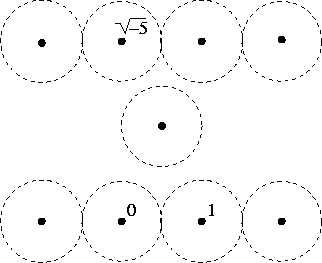Thus there exist points in the complex plane with distance $$\ge 1$$ from the nearest Kummer integer, so Euclid's proof of unique prime factorization fails — and so does unique prime factorization: $2 \cdot 3 = 6 = (1 + \sqrt{-5})(1 - \sqrt{-5}) .$

In short, there is an interesting relation between number theory and a subject on which Conway is an expert: densely packed lattices . For a lattice in a normed division algebra to be closed under multiplication, all its points must have distance $$\ge 1$$ from each other: otherwise the smallest element of nonzero norm, say $$z$$, would have $$|z| < 1$$ and thus $$|z^2| < |z|$$ — a contradiction! On the other hand, for the Euclidean algorithm to work, at least in the simple form described here, there must be no point in the plane with distance $$\ge 1$$ from the nearest lattice point. So for both of these to hold, our lattice must be 'well packed': if we place open balls of radius $$1/2$$ centered at all the lattice points, they must be disjoint, but they must not leave room for any more disjoint open balls of this radius.

When it comes to subrings of the complex numbers, these ideas are well-known. Back in the 1890's, Minkowski used them to study unique prime factorization (or more generally, ideal class groups) not only for algebraic integers in quadratic number fields, as we have secretly been doing here, but also in other number fields, which require lattices in higher dimensions. He called this subject 'the geometry of numbers' [4, 11, 16]. Conway and Smith explore a lesser-known aspect of the geometry of numbers by applying it to subrings of the quaternions and octonions. But they cannot resist a little preliminary detour into the geometry of lattices in 2 dimensions — nor should we.

The Gaussian and Eisenstein integers are the most symmetrical lattices in the plane, since they have 4-fold and 6-fold rotational symmetry, respectively. As such, they naturally turn up in the classification of 2-dimensional space groups. A 'space group' is a subgroup of the Euclidean group (the group of transformations of $$\mathbb{R}^n$$ generated by rotations, reflections and translations) that acts transitively on a lattice. Up to isomorphism, there are 230 space groups in 3 dimensions. These act as symmetries of various kinds of crystals, so they form a useful classification scheme in crystallography — perhaps the most easily understood application of group theory to physics. In 2 dimensions, there are just 17 isomorphism classes of space groups. These are also called 'wallpaper groups', since they act as symmetries of different wallpaper patterns. Conway and Smith describe all these groups. Two of them act on a lattice with the least amount of symmetry: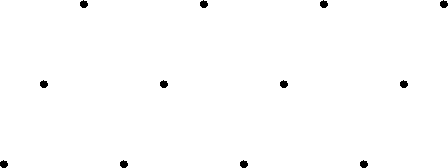Seven act on a lattice with rectangular symmetry: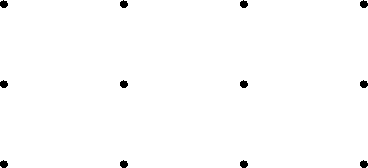or alternatively, on one with rhombic symmetry. Three act on a lattice with square symmetry, and five act on a lattice with hexagonal symmetry.

After this low-dimensional warmup, Conway and Smith's book turns to the quaternions and their applications to geometry. The quaternions were discovered by Sir William Rowan Hamilton in 1843. Fascinated by the applications of complex numbers to 2d geometry, he had been struggling unsuccessfully for many years to invent a bigger algebra that would do something similar for 3d geometry. In modern language, it seems he was looking for a 3-dimensional normed division algebra. Unfortunately, no such thing exists! Finally, on October 16th, while walking with his wife along the Royal Canal to a meeting of the Royal Irish Academy in Dublin, he discovered a 4-dimensional normed division algebra. In his own words, "I then and there felt the galvanic circuit of thought close; and the sparks which fell from it were the fundamental equations between $$i, j, k$$; exactly such as I have used them ever since." He was so excited that he carved these equations on the soft stone wall of Brougham Bridge.

Hamilton's original inscription has long since been covered by other graffiti, though a plaque remains to commemorate the event. The quaternions, which in the late 1800's were a mandatory examination topic in Dublin and the only advanced mathematics taught in some American universities, have now sunk into obscurity. The reason is that the geometry and physics which Hamilton and his followers did with quaternions is now mostly done using the dot product and cross product of vectors, invented by Gibbs in the 1880's . Scott Kim's charming sepia-toned cover art for this book nicely captures the 'old-fashioned' flavor of some work on quaternions. But the quaternions are also crucial to some distinctly modern mathematics and physics.

As a vector space, the quaternions are $\mathbb{H} = \{ a + bi + cj + dk \; \colon \; a,b,c,d \in \mathbb{R} \} .$ They become an associative algebra with 1 as the multiplicative unit via: $i^2 = j^2 = k^2 = -1,$ $ij = k = -ji {\rm \; and \; cyclic \; permutations}.$ Copying what works for complex numbers, we define the 'conjugate' of a quaternion $$q = a + bi + cj + dk$$ to be $$\overline{q} = a - bi - cj - dk$$, and define its 'real part' to be $$\mathrm{Re}(q) = a$$. It is then easy to check that $q \overline{q} = \overline{q} q = a^2 + b^2 + c^2 + d^2 .$ This suggests defining a norm by $$|q|^2 = q \overline{q}$$, and it then turns out that the quaternions are a normed division algebra: $|qq'| = |q| |q'| .$ In particular, any nonzero quaternion has a two-sided multiplicative inverse given by $q^{-1} = \overline{q} / |q|^2 .$

It follows that the quaternions of norm 1 form a group under multiplication. This group is usually called $$\mathrm{SU}(2)$$, because people think of its elements as $$2 \times 2$$ unitary matrices with determinant 1. However, the quaternionic viewpoint is better adapted to seeing how this group describes rotations in 3 and 4 dimensions. The unit quaternions act via conjugation as rotations of the 3d space of 'pure imaginary' quaternions, namely those with $$\mathrm{Re}(q) = 0$$. This gives a homomorphism from $$\mathrm{SU}(2)$$ onto the 3d rotation group $$\mathrm{SO}(3)$$. The kernel of this homomorphism is $$\{ \pm 1 \}$$, so we see $$\mathrm{SU}(2)$$ is a double cover of $$\mathrm{SO}(3)$$. The unit quaternions also act via left and right multiplication as rotations of the 4d space of all quaternions. This gives a homomorphism from $$\mathrm{SU}(2) \times \mathrm{SU}(2)$$ onto the 4d rotation group $$\mathrm{SO}(4)$$. The kernel of this homomorphism is $$\{ \pm (1,1) \}$$, so we see $$\mathrm{SU}(2) \times \mathrm{SU}(2)$$ is a double cover of $$\mathrm{SO}(4)$$.

These facts are incredibly important throughout mathematics and physics. With their help, Conway and Smith classify the finite subgroups of the 3d rotation group $$\mathrm{SO}(3)$$, its double cover $$\mathrm{SU}(2)$$, the 3d rotation/reflection group $$\mathrm{O}(3)$$, and the 4d rotation group $$\mathrm{SO}(4)$$. These classifications are all in principle 'well known'. However, they seem hard to find in one place, so Conway and Smith's elegant treatment is very helpful.

Next, Conway and Smith turn to quaternionic number theory. The obvious analogue of the Gaussian integers are the 'Lipschitz integers', namely quaternions of the form $$a + bi + cj + dk$$ where $$a,b,c,d$$ are all integers. The Lipschitz integers are a subring of the quaternions, and this has a nice application to ordinary number theory. Applying the formula $$|zz'| = |z||z'|$$ to the product of two Gaussian integers gives the famous 'two squares formula': $(x^2 + y^2)(x'^2 + y'^2) = (xx' - yy')^2 + (xy' + yx')^2$ which shows that the set of integers expressible as the sum of two squares is closed under multiplication. Similarly, taking the norm of the product of two Lipschitz integers gives a 'four squares formula'. This shows the set of integers expressible as the sum of four squares is closed under multiplication. This fact is less impressive than it might at first sound, since one can prove that all integers can be written as the sum of four squares — but the four-square formula reduces the task of proving this to the case of prime numbers.

Alas, the Lipschitz integers are not well packed, so their factorization into Lipschitz primes is far from unique. This was noted already by Lipschitz himself . For example: $(1 + i)(1 - i) = 2 = (1 + j)(1 - j).$ However, it is easy to correct this problem. Consider the cubical lattice of all points with integer coordinates in $$\mathbb{R}^n$$. To make the distance to the nearest lattice point as big as possible, we can go to any point with all half-integer coordinates. The distance to the nearest lattice point is then $\sqrt{\left(\frac{1}{2}\right)^2 + \cdots + \left(\frac{1}{2}\right)^2 } = \textstyle{\sqrt{n/4}}.$ This gets arbitrarily large as $$n$$ increases — so in high dimensions we could pack space with a cubical lattice of steel balls with half-inch radius snugly touching each other, but still leave places light-years away from metal.

More to the point, the distance $$\sqrt{n/4}$$ reaches $$1$$ precisely in dimension $$4$$ — the case we are interested in. So, if we place open balls of radius $$1/2$$ centered at the Lipschitz integers, there is still room to slip in a translated copy of this lattice of balls centered at quaternions $$a + bi + cj + dk$$ where $$a,b,c,d$$ are half-integers. This gives the 'Hurwitz integers': quaternions of the form $$a + bi + cj + dk$$ where $$a,b,c,d$$ are either all integers or all integers plus $$1/2$$.

The Hurwitz integers are a well-packed lattice and also a subring of the quaternions. This lets Conway and Smith prove a version of unique prime factorization for Hurwitz integers. To state this result, they restrict attention to 'primitive' Hurwitz integers, namely those that are not divisible by any natural number. They show that for any primitive Hurwitz integer $$Q$$ and any factorization of $$|Q|^2$$ into a product $$p_0 p_1 \cdots p_k$$ of ordinary prime numbers, there is a factorization $Q = P_0 P_1 \cdots P_k$ of $$Q$$ into a product of Hurwitz primes with $$|P_i|^2 = p_i$$. Moreover, given any factorization with this property, all the other factorizations with this property are of the form $Q = (P_0U_1) (U_1^{-1}P_1U_2) \cdots (U_k^{-1}P_k)$ where the $$U_i$$ are Hurwitz integers of norm 1 — of which there are precisely 24, as we shall soon see. Conway and Smith call this 'uniqueness up to unit-migration'. For example, these two factorizations are not the same up to unit-migration if we work in the Lipschitz integers: $(1 + i)(1 - i) = 2 = (1 + j)(1 - j)$ but they become so in the Hurwitz integers, since we have $(1 + i)U = (1 + j), \qquad U^{-1}(1 - i) = (1 - j)$ where $$U$$ is a Hurwitz integer of norm 1, namely $$\frac{1}{2}(1 - i + j - k)$$.

The Hurwitz integers are so beautiful that we should pause and admire them before following Conway and Smith to higher dimensions. Though it is far from obvious, they give the densest possible lattice packing of balls in 4 dimensions . In this setup, each ball touches 24 others. For example, the ball centered at the origin touches the balls centered at Hurwitz integers of norm 1. There are 8 of these with integer coordinates: $\pm 1, \; \pm i, \; \pm j, \; \pm k,$ and 16 with half-integer coordinates: $\frac{1}{2}(\pm 1 \pm i \pm j \pm k ) .$ The 8 with integer coordinates form the vertices of a cross-polytope (the 4d analogue of an octahedron):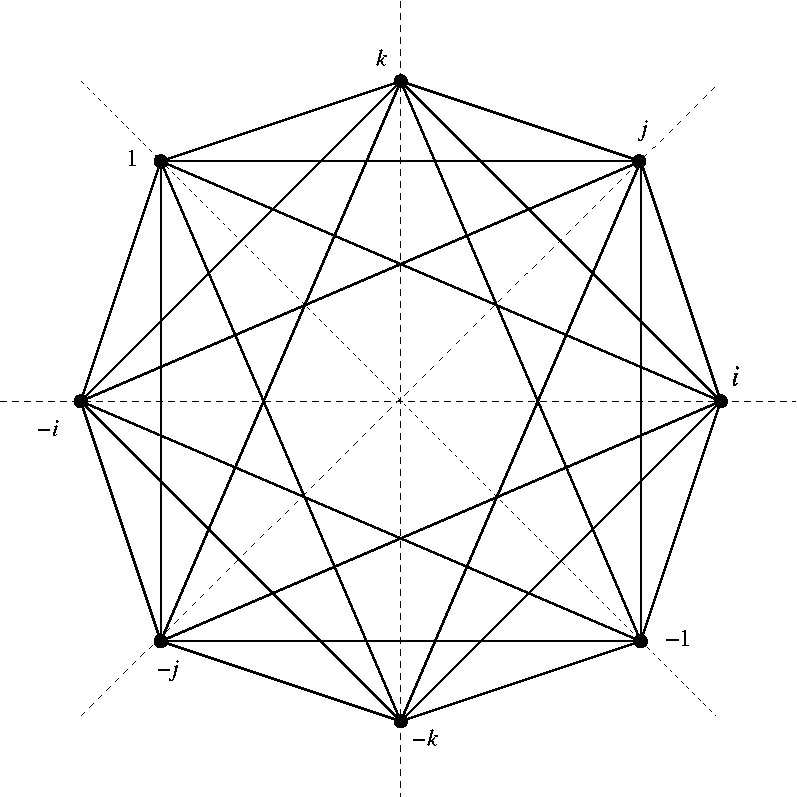while the 16 with half-integer coordinates form the vertices of a hypercube (the 4d analogue of a cube):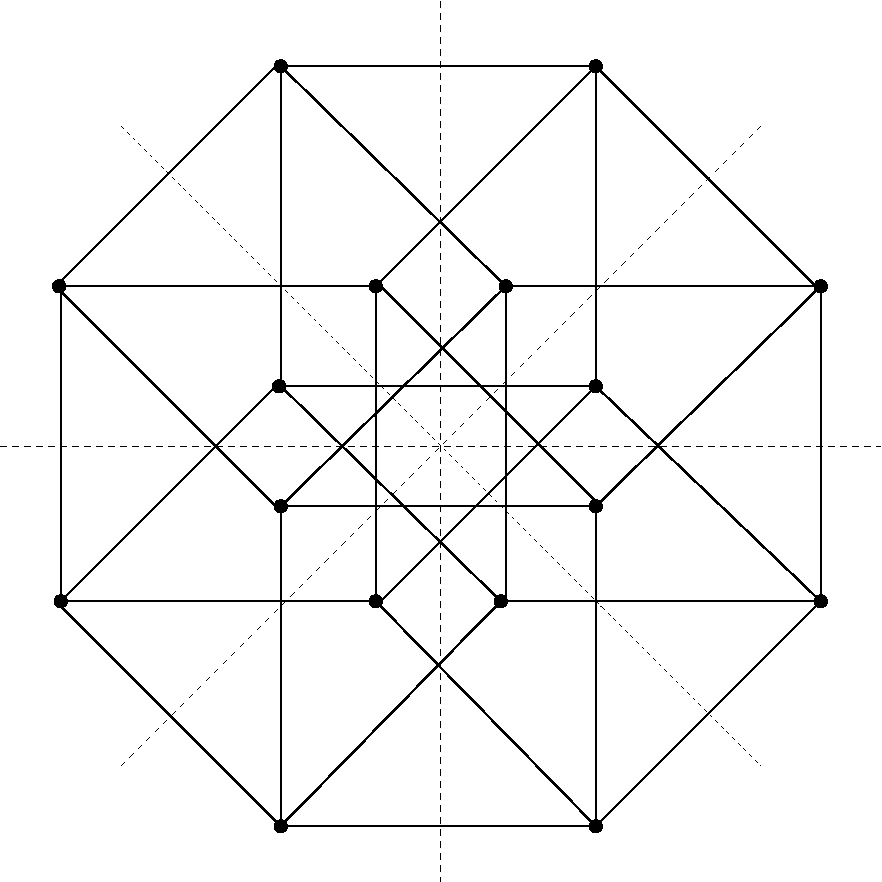Taken together, they form the vertices of a regular polytope called the '24-cell':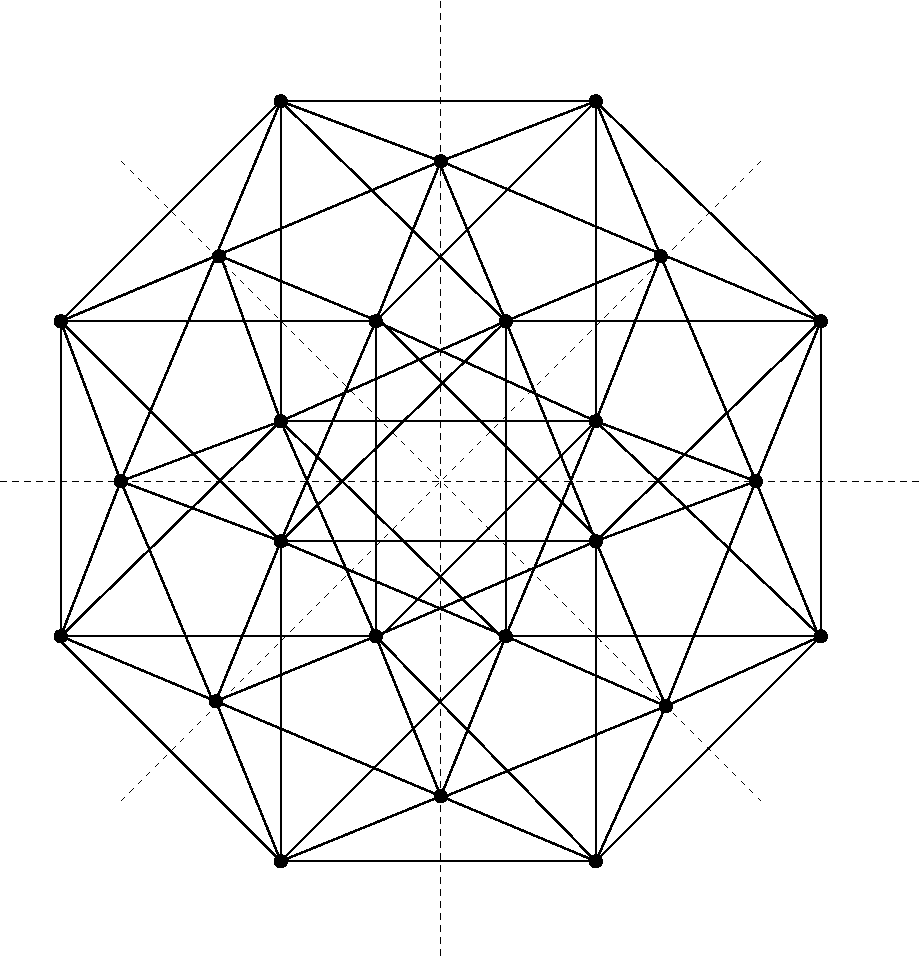All but one of the regular polytopes in 4 dimensions are analogues of Platonic solids in 3 dimensions; the exception is the 24-cell. The picture above is a bit too cluttered to reveal all the charms of this entity. It is helpful to look at 3-dimensional slices: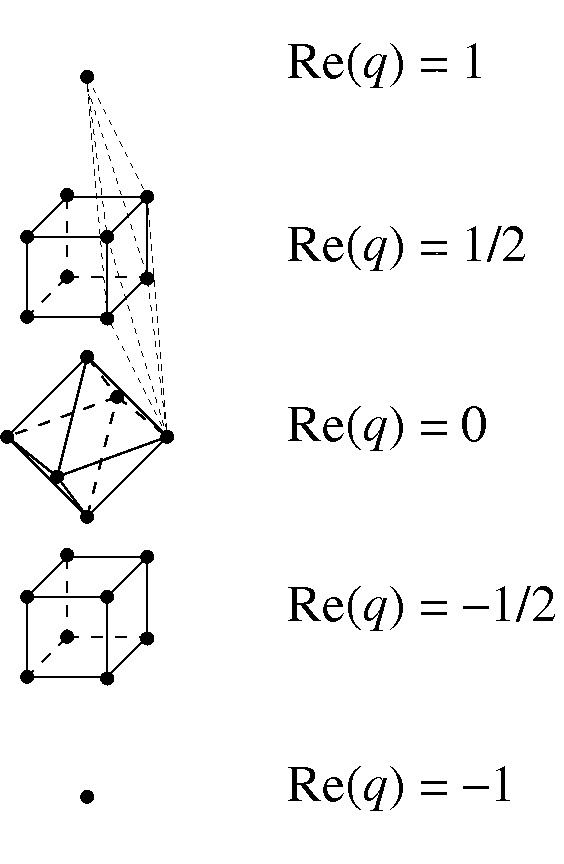The thin dashed lines show one of the faces of the 24-cell: though distorted in this picture, it is really a regular octahedron. Since the hypercube is dual to the cross-polytope, the 24-cell is self-dual — so it has 24 of these octahedral faces, and if we draw a dot in the middle of each one, we get the vertices of another 24-cell.

There is much more to say in favor of the 24-cell. It is not only a regular polytope; it is also a group! More precisely, its vertices form a 24-element subgroup of $$\mathrm{SU}(2)$$. This is usually called the 'binary tetrahedral group', since it is a double cover of the rotational symmetry group of the tetrahedron. This group also goes by the name of $$\mathrm{SL}(2,\mathbb{Z}/3)$$: $$2 \times 2$$ matrices with determinant 1 having entries in the integers modulo 3. In this guise it explains some of the mystical importance of the number 24 in bosonic string theory .

It would be enjoyable to spend more time delving into these matters, but alas, this review is not the proper place. Instead, we should move on to the octonions. These were first discovered by Hamilton's college friend John Graves. It had been Graves' interest in algebra that got Hamilton thinking about complex numbers and their generalizations in the first place. The day after discovering the quaternions, Hamilton sent a letter describing them to Graves. The day after Christmas on that same year, Graves wrote to Hamilton describing an 8-dimensional algebra which he called the 'octaves'. He showed that they were a normed division algebra, and used this to express the product of two sums of eight squares as another sum of eight squares: the 'eight squares theorem'. Hamilton offered to publicize Graves' discovery, but kept putting it off, absorbed in work on the quaternions. Eventually Arthur Cayley rediscovered them and published an article announcing their existence in 1845. For this reason they are sometimes called 'Cayley numbers' — but these days, all right-thinking people call them the 'octonions'.

As a vector space, the octonions are $\mathbb{O} = \{ a_0 + \sum_{i = 1}^7 a_i e_i \; \colon \; a_0, \dots, a_7 \in \mathbb{R} \} .$ We make them into a nonassociative algebra with $$1$$ as multiplicative unit using a gadget called the 'Fano plane':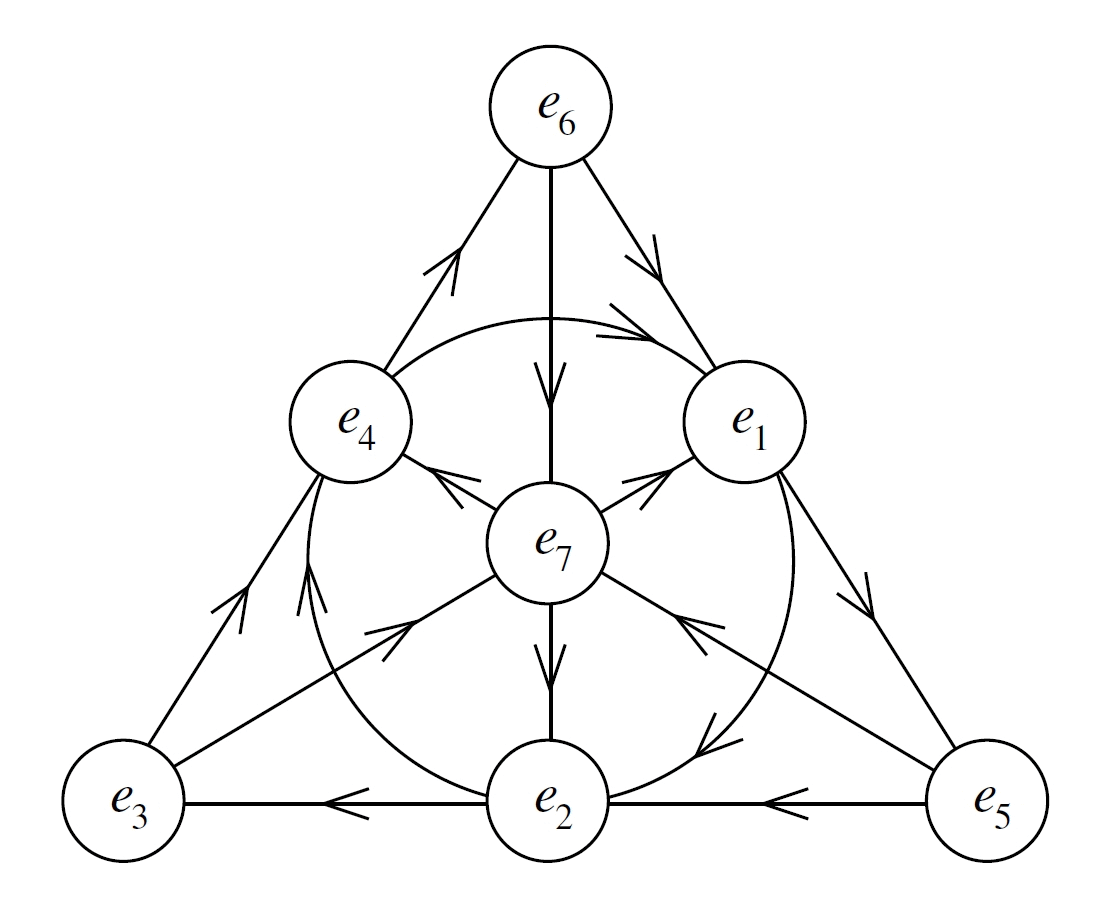There are 7 points and 7 lines in this picture, if we count the circle containing $$e_1,e_2,e_4$$ as an honorary 'line'. Each line contains 3 points, and each of these triples is equipped with a cyclic order as indicated by the arrows. The rule is that if $$e_i, e_j, e_k$$ are cyclically ordered in this way, they satisfy: $e_i^2 = e_j^2 = e_k^2 = -1,$ $e_i e_j = e_k = -e_j e_i {\rm \; and \; cyclic \; permutations}.$ Thus, they give a copy of the quaternions inside the octonions.

Copying what worked for the quaternions, we define the 'conjugate' of an octonion $$a = a_0 + \sum_{i=1}^7 a_i e_i$$ to be $$\overline{a} = a_0 - \sum_{i=1}^7 a_i e_i$$, and define its 'real part' to be $$\mathrm{Re}(a) = a_0$$. It is then easy to check that $a \overline{a} = \overline{a} a = \sum_{i=0}^7 a_i^2 ,$ so we can define a norm by $$|a|^2 = a \overline{a}$$. This makes the octonions into a normed division algebra: $|aa'| = |a| |a'| ,$ and any nonzero octonion has a two-sided inverse given by $a^{-1} = \overline{a} / |a|^2 .$ A brute-force verification of these last facts is unpleasant, in part because the octonions are nonassociative. It is also not very enlightening. Conway and Smith wisely use the 'Cayley–Dickson construction' instead. This is a dimension-doubling procedure that produces $$\mathbb{C}$$ from $$\mathbb{R}$$, $$\mathbb{H}$$ from $$\mathbb{C}$$, and $$\mathbb{O}$$ from $$\mathbb{H}$$, and explains why they are normed division algebras — while also explaining why there are no more.

Conway and Smith then develop the fascinating relationship between octonions and $$\mathrm{Spin}(8)$$, the double cover of the rotation group in 8 dimensions. In physics lingo, the octonions can be described not only as the vector representation of $$\mathrm{Spin}(8)$$, but also the left-handed spinor representation and the right-handed spinor representation. This fact is called 'triality'. It has many amazing spinoffs, including structures like the exceptional Lie groups and the exceptional Jordan algebra, and the fact that supersymmetric string theory works best in 10-dimensional spacetime — fundamentally because the 2-dimensional worldsheet of the string wants 8 extra dimensions to wiggle around in, and $$8 + 2 = 10$$. To develop the theory of triality, Conway and Smith make use of Moufang loops and their isotopies — two concepts which never made much sense to me until I saw their lucid treatment. Anyone interested in triality must read this section.

Next, Conway and Smith tackle octonionic number theory. Various lattices in $$\mathbb{O}$$ present themselves as possible octonionic analogues of the integers, but the best candidate is the least obvious. Starting with the most obvious, the 'Gravesian integers' are octonions of the form $a = a_0 + \sum_{i=1}^7 a_i e_i$ where all the coefficients $$a_i$$ are integers. The 'Kleinian integers' are octonions where the $$a_i$$ are either all integers or all half-integers. Both these are lattices closed under multiplication — but alas, neither is well-packed. To get a denser lattice, first pick a line in the Fano plane. Then, take all integral linear combinations of Gravesian integers, octonions of the form $\frac{1}{2}(\pm 1 \pm e_i \pm e_j \pm e_k)$ where $$e_i$$, $$e_j$$ and $$e_k$$ lie on this line, and those of the form $\frac{1}{2}( \pm e_p \pm e_q \pm e_r \pm e_s)$ where $$e_p, e_q, e_r, e_s$$ all lie off this line. The resulting lattice is called the 'double Hurwitzian integers'. Actually we obtain 7 isomorphic copies of the double Hurwitzian integers this way, one for each line in the Fano plane.

The double Hurwitzian integers are closed under multiplication, and it is easy to see that as a lattice, they are the product of two copies of the Hurwitz integers — hence their name. In fact, they can be obtained from the Hurwitz integers using the Cayley–Dickson doubling construction. But unlike the Hurwitz integers, they are not well-packed. To see this, note that the point $\frac{1}{2}(1 + e_i + e_p + e_q)$ has distance 1 from all the double Hurwitzian integers.

To fix this, we need an even denser lattice closed under multiplication. One natural guess is to take the union of all 7 copies of the double Hurwitzian integers. This gives a well-packed lattice — and in fact, the densest possible lattice packing of balls in 8 dimensions. In this setup, each ball touches 240 others. To see this, just count the lattice vectors of norm 1. First, we have $$\pm e_i$$ for $$i=0, \dots 7$$. Second, we have $$\frac{1}{2}(\pm 1 \pm e_i \pm e_j \pm e_k)$$ where $$e_i$$, $$e_j$$ and $$e_k$$ all lie on some line in the Fano plane. And third, we have $$\frac{1}{2}( \pm e_p \pm e_q \pm e_r \pm e_s)$$ where $$e_p, e_q, e_r, e_s$$ all lie off some line. There are $$2 \times 8 = 16$$ vectors of the first form, $$2^4 \times 7 = 112$$ of the second form, and $$2^4 \times 7 = 112$$ of the third form, for a total of 240.

Curiously, I had just been thinking about this lattice when Conway and Smith's book arrived in my mail. After checking a couple of cases, I had jumped to the conclusion that it is closed under multiplication. I was shocked to read that it is not. But I was comforted to hear that this is a common mistake. Following Coxeter , Conway and Smith call it 'Kirmse's mistake', after the first person to make it in public. To rub salt in the wound, they mockingly call this lattice the 'Kirmse integers'.

To fix Kirmse's mistake, you need to perform a curious trick. Pick a number $$j$$ from 1 to 7. Then, take all the Kirmse integers $a = a_0 + \sum_{i=1}^7 a_i e_i$ and switch the coefficients $$a_0$$ and $$a_j$$. As a lattice, the resulting 'Cayley integers' are just a reflected version of the Kirmse integers, so they are still well-packed. But bizarrely, they are now closed under multiplication! Since this trick involved an arbitrary choice, there are 7 different copies of the Cayley integers containing the Gravesian integers. And this is as good as it gets: each one is maximal among lattices closed under multiplication.

Conway and Smith then study prime factorization in the Cayley integers. This is a fascinating subject, but even trickier than the quaternionic case, since the octonions are nonassociative: one has to worry about different parenthesizations, as well as different orderings. So at this point, I will stop trying to explain their work, and leave it to them. Instead, I will say a bit about a topic that Conway and Smith skip: how the Hurwitz integers and Cayley integers show up in the theory of Lie groups. Every compact simple Lie group $$K$$ has a subgroup that is isomorphic to a product of circles and is as big as possible while having this property. Though not unique, this subgroup is unique up to conjugation; it is called a 'maximal torus' and denoted $$T$$. Since it is abelian, it is much easier to study than $$K$$ itself. We cannot recover $$K$$ just from this subgroup $$T$$. But $$K$$ has a god-given Riemannian metric on it, which restricts to a metric on $$T$$. One of the miracles of Lie theory is that knowing the group $$T$$ together with this metric on it is enough to determine $$K$$ up to isomorphism, at least when $$K$$ is connected.

We can simplify things even further if we work with the Lie algebra $$\mathfrak{t}$$ of the torus $$T$$. Since $$T$$ is abelian, the bracket on $$\mathfrak{t}$$ vanishes. If that were all, $$\mathfrak{t}$$ would be a mere vector space. However, $$\mathfrak{t}$$ also has an inner product coming from the Riemannian metric on $$T$$. There is also a lattice $$L$$ in $$\mathfrak{t}$$, namely the kernel of the exponential map $\exp \colon \mathfrak{t} \to T .$ So, $$\mathfrak{t}$$ is really an inner product space with a lattice in it. From the lattice one can recover the torus $$T \cong \mathfrak{t}/L$$, and from the inner product on $$\mathfrak{t}$$ one can recover the metric on this torus. So, we have compressed all the information about $$K$$ into something very simple: an inner product space containing a lattice. Not any lattice in an inner product space comes from a compact simple Lie group in this manner. However, we can work out which ones do — and Hurwitz integers and the Cayley integers do!

In this context, the Hurwitz integers are called the '$$\mathrm{D}_4$$ lattice', and the corresponding Lie group is $$\mathrm{Spin}(8)$$, the double cover of the rotation group in 8 dimensions. I already mentioned that this group is closely tied to the octonions via triality. Now we are seeing its ties to the quaternions! In this context, triality manifests itself as the symmetry that cyclically permutes the Hurwitz integers $$i, j,$$ and $$k$$.

Similarly, in this context the Cayley integers are called the '$$\mathrm{E}_8$$ lattice'. The corresponding group is also called $$\mathrm{E}_8$$. It is the biggest of the 5 exceptional cases that show up in the classification of compact simple Lie groups. In order of increasing dimension, these are called $$\mathrm{G}_2, \mathrm{F}_4, \mathrm{E}_6, \mathrm{E}_7$$ and $$\mathrm{E}_8$$ — where the subscript gives the dimension of the maximal torus. They are all connected to the octonions, and they all play a role in string theory. In some ways $$\mathrm{E}_8$$ is the most mysterious, because its smallest nontrivial representation is the 'adjoint representation', in which it acts by conjugation on its own Lie algebra. Since $$\mathrm{E}_8$$ is 248-dimensional, this means that the smallest matrices we can use to describe its elements are of size $$248 \times 248$$. This is a nuisance, but the real problem is that the best way to understand a group is to see it as the group of symmetries of something. In the adjoint representation, we are only seeing $$\mathrm{E}_8$$ as symmetries of itself! It seems to be pulling itself up into existence by its own bootstraps.

Recently some mathematical physicists have been studying a construction of $$\mathrm{E}_8$$ as the symmetries of a 57-dimensional complex manifold equipped with extra structure [12,14]. When I heard this, the number 57 instantly intrigued me — and not just because Heinz advertises 57 varieties of ketchup, either. No, the real reason was that the smallest nontrivial representation of $$\mathrm{E}_8$$'s little brother $$\mathrm{E}_7$$ is 56-dimensional. When you study exceptional Lie algebras, you start noticing that strange numbers can serve as clues to hidden relationships... and indeed, there is one here.

One can actually find the numbers 56 and 57 lurking in the geometry of the 240 Cayley integers of norm 1. However, it helps to begin with some general facts about graded Lie algebras. Here I am not referring to $$\mathbb{Z}/2$$-graded Lie algebras, also known as 'Lie superalgebras'. Instead, I mean Lie algebras $$\mathfrak{g}$$ that have been written as a direct sum of subspaces $$\mathfrak{g}(i)$$, one for each integer $$i$$, such that $$[\mathfrak{g}(i), \mathfrak{g}(j)] \subseteq \mathfrak{g}(i+j)$$. If only the middle 3 of these subspaces are nonzero, so that $\mathfrak{g} = \mathfrak{g}(-1) \oplus \mathfrak{g}(0) \oplus \mathfrak{g}(1) ,$ we say that $$\mathfrak{g}$$ is '3-graded'. Similarly, if only the middle 5 are nonzero, so that $\mathfrak{g} = \mathfrak{g}(-2) \oplus \mathfrak{g}(-1) \oplus \mathfrak{g} (0) \oplus \mathfrak{g}(1) \oplus \mathfrak{g}(2) ,$ we say L is '5-graded', and so on. In these situations, some nice things happen . First of all, $$\mathfrak{g}(0)$$ is always a Lie subalgebra of $$\mathfrak{g}$$. Second of all, it acts on each other space $$\mathfrak{g}(i)$$ by means of the bracket. Third of all, if $$\mathfrak{g}$$ is 3-graded, we can give $$\mathfrak{g}(1)$$ a product by picking any element $$k \in \mathfrak{g}(-1)$$ and defining $x \circ y = [[x,k],y] .$ This product automatically satisfies the identities defining a 'Jordan algebra': $x \circ y = y \circ x ,$ $x \circ (y \circ (x \circ x)) = (x \circ y) \circ (x \circ x) ,$ so 3-graded Lie algebras are a great source of Jordan algebras .

If $$\mathfrak{k}$$ is the Lie algebra of a compact simple Lie group $$K$$, there is a very nice way to look for gradings of its complexification $$\mathfrak{g} = \mathbb{C} \otimes \mathfrak{k}$$. This involves some more Lie theory — standard stuff that I will only briefly sketch here [1, 10]. Recall that we can pick a maximal torus $$T$$ for $$K$$. The Lie algebra $$\mathfrak{t}$$ of this maximal torus is contained in $$\mathfrak{k}$$, and similarly its complexification $$\mathfrak{h} = \mathbb{C} \otimes \mathfrak{t}$$ is contained in $$\mathfrak{g}$$. It turns out that $$\mathfrak{g}$$ is the direct sum of $$\mathfrak{h}$$ and a bunch of 1-dimensional complex vector spaces $$\mathfrak{g}_r$$, one for each 'root' $$r$$. Roots are certain special vectors in the 'dual lattice' $$L^\ast$$, meaning the lattice of vectors $$\ell \in \mathfrak{t}^\ast$$ such that $$\ell(v)$$ is an integer for all $$v$$ in the original lattice $$L$$. It is handy to define $$\mathfrak{g}_0$$ to be $$\mathfrak{h}$$, so that $\mathfrak{g} = \bigoplus_{r \in \{{\rm roots}\} \cup \{0\}} \mathfrak{g}_r .$ The great thing about this decomposition is that $[\mathfrak{g}_r , \mathfrak{g}_{r'} ] \subseteq \mathfrak{g}_{r + r'}$ whenever $$r$$ and $$r'$$ are either roots or zero. So, to put a grading on $$\mathfrak{g}$$, we just need to slice $$\mathfrak{t}$$ with evenly spaced parallel hyperplanes in such a way that each root, as well as the origin, lies on one of these hyperplanes.

Now let us turn to the case of $$\mathrm{E}_8$$. Let us call the complexification of its Lie algebra — what we have been calling $$\mathfrak{g}$$ above — simply $$\mathfrak{e}_8$$. In this case $$\mathfrak{t}$$ is the octonions and $$L$$ is the Cayley integers. However, it will be simpler to work in a coordinate system where $$L$$ is the Kirmse integers, since they have the same geometry as a lattice, and they are easier to describe. This could be called 'Kirmse's revenge'.

If we use the inner product $$\langle x,y \rangle = \mathrm{Re}(x^\ast y)$$ on the octonions to identify $$\mathfrak{t}$$ with its dual, it turns out that $$L^\ast = L$$: the lattice of Kirmse integers is self-dual. Moreover, the roots are just the Kirmse integers of norm 1. Since there are 240 of these, the dimension of $$\mathrm{E}_8$$ is $$240 + \dim(\mathfrak{h}) = 248$$.

To put a grading on $$\mathfrak{e}_8$$, you should imagine these 240 roots as the vertices of a gleaming 8-dimensional diamond. Imagine yourself as a gem cutter, turning around this diamond, looking for nice ways to slice it. You need to slice it with evenly spaced parallel hyperplanes that go through every vertex, as well as the center of the diamond.

The easiest way to do this is to let each slice go through all the roots whose real part takes a given value. This value can be $$1, \frac{1}{2}, 0, -\frac{1}{2},$$ or $$-1$$, so we obtain a 5-grading of the Lie algebra $$\mathfrak{e}_8$$. We can count the number of roots in each slice:

• The number of roots with real part 1 is 1.
• The number of roots with real part $$\frac{1}{2}$$ is 56.
• The number of roots with real part 0 is 126.
• The number of roots with real part $$-\frac{1}{2}$$ is 56.
• The number of roots with real part $$-1$$ is 1.
The only root with real part 1 is the octonion 1. Similarly, the only root with real part $$-1$$ is the octonion $$-1$$. We get 56 roots with real part $$\frac{1}{2}$$ by multiplying the number of lines in the Fano plane by the number of sign choices in $\frac{1}{2}(1 \pm e_i \pm e_j \pm e_k) .$ The number of roots with real part $$-\frac{1}{2}$$ is the same, by symmetry. We get 126 roots with real part 0 by subtracting all the other numbers on the above list from 240.

It follows that there is a 5-grading of $$\mathfrak{e}_8$$: $\mathfrak{e}_8 = \mathfrak{e}_8(-2) \oplus \mathfrak{e}_8(-1) \oplus \mathfrak{e}_8(0) \oplus \mathfrak{e}_8(1) \oplus \mathfrak{e}_8(2) ,$ where the dimensions of the subspaces work as follows: $248 = 1 + 56 + 134 + 56 + 1 .$ Here we must remember to include $$\mathfrak{t}$$ in $$\mathfrak{e}_8(0)$$, obtaining a Lie subalgebra of dimension $$126 + 8 = 134$$.

This immediately shows how to get $$\mathrm{E}_8$$ to act on a 57-dimensional manifold. Form the group $$\mathrm{E}_8$$, and form the subgroup $$P$$ whose Lie algebra is $$\mathfrak{e}_8(-2) \oplus \mathfrak{e}_8(-1) \oplus \mathfrak{e}_8(0)$$. The quotient $$\mathrm{E}_8/P$$ is a manifold on which $$\mathrm{E}_8$$ acts. Its tangent spaces all look like $$\mathfrak{e}_8(1) \oplus \mathfrak{e}_8(2)$$, so they are 57-dimensional! These tangent spaces are complex vector spaces, so we are getting a 57-dimensional complex manifold on which the complexification of $$\mathrm{E}_8$$ acts, but with some extra work we can get certain 'real forms' of $$\mathrm{E}_8$$ to act on 57-dimensional real manifolds. The options have been catalogued by Kaneyuki .

In the above grading of $$\mathfrak{e}_8$$, the 134-dimensional Lie algebra $$\mathfrak{e}_8(0)$$ is the direct sum of the Lie algebra $$\mathfrak{e}_7$$ and the 1-dimensional abelian Lie algebra $$\mathfrak{gl}(1)$$. This comes as little surprise if one knows that the dimension of $$\mathfrak{e}_7$$ is 133, but the reason for it is that if we take all the roots of $$\mathfrak{e}_8$$ that are orthogonal to a given root, we obtain the roots of $$\mathfrak{e}_7$$. From this point of view, the 5-grading of $$\mathfrak{e}_8$$ looks like this: $\mathfrak{e}_8 = \mathbb{C} \oplus \mathbb{F} \oplus (\mathfrak{e}_7 \oplus \mathfrak{gl}(1)) \oplus \mathbb{F} \oplus \mathbb{C} .$ Recall that $$\mathfrak{e}_8(0) = \mathfrak{e}_7 \oplus \mathfrak{gl}(1)$$ acts on all the other spaces $$\mathfrak{e}_8(i)$$. In particular, $$\mathbb{C}$$ is the 1-dimensional trivial representation of $$\mathfrak{e}_7 \oplus \mathfrak{gl}(1)$$, while $$\mathbb{F}$$ is the 'Freudenthal algebra': a 56-dimensional representation of $$\mathfrak{e}_7 \oplus \mathfrak{gl}(1)$$, which happens to be the smallest nontrivial representation of $$\mathfrak{e}_7$$. This gadget was Freudenthal's way of trying to understand the group $$\mathrm{E}_7$$. It has a symplectic structure and ternary product that are invariant under $$\mathrm{E}_7$$, and he showed that $$\mathrm{E}_7$$ is precisely the group of transformations preserving these structures [2,9]. It is not clear to me how enlightening this is. More interesting is that these three facts:

• $$\mathrm{E}_8$$ is 248-dimensional.
• $$\mathrm{E}_8$$ is the symmetry group of a 57-dimensional complex manifold equipped with extra geometrical structure.
• $$\mathrm{E}_7$$ is the symmetry group of a 56-dimensional vector space equipped with extra algebraic structure.

turn out to have a common origin: namely, that when we pack 8-dimensional balls in a lattice modelled after the Cayley integers, each ball has 240 nearest neighbors, and when we take any one of these neighbors and count the number of others that touch it, we find that there are 56!

There are many more games to play along these lines. For example, we have just seen that the pure imaginary Kirmse integers of norm 1 are the roots of $$\mathrm{E}_7$$. These form the vertices of a gemstone in 7 dimensions, and we can repeat our 'gem-cutting' trick to get a 3-grading of $$\mathrm{E}_7$$: $\mathfrak{e}_7 = \mathfrak{e}_7(-1) \oplus \mathfrak{e}_7(0) \oplus \mathfrak{e}_7(1)$ for which the dimensions work as follows: $133 = 27 + 79 + 27 .$ Since the dimension of $$\mathfrak{e}_6$$ is 78, it is not very surprising that $$\mathfrak{e}_7(0)$$ is the direct sum of $$\mathfrak{e}_6$$ and the one-dimensional abelian Lie algebra $$\mathfrak{gl}(1)$$. Since 3-gradings give Jordan algebras, it is also not surprising that $$\mathfrak{e}_7(1)$$ is a famous 27-dimensional Jordan algebra. The 'exceptional Jordan algebra' is the space $$\mathfrak{h}_3(\mathbb{O})$$ of $$3 \times 3$$ self-adjoint matrices with octonion entries, equipped with the product $$a \circ b = \frac{1}{2}(ab + ba)$$. This is a Jordan algebra over the real numbers. It is 27-dimensional, since its elements look like this: $\mathfrak{h}_3(\mathbb{O}) = \{ \left( \begin{array}{ccc} \alpha & z^* & y^* \\ z & \beta & x \\ y & x^* & \gamma \end{array} \right) : \; x,y,z \in \mathbb{O} , \; \alpha , \beta, \gamma \in \mathbb{R} \} .$ Its complexification $$\mathbb{J} = \mathbb{C} \otimes \mathfrak{h}_3(\mathbb{O})$$ is none other than $$\mathfrak{e}_7(1)$$. The space $$\mathfrak{e}_7(-1)$$ is best thought of as the dual of this, so we have: $\mathfrak{e}_7 = \mathbb{J}^\ast \oplus (\mathfrak{e}_6 \oplus \mathfrak{gl}(1)) \oplus \mathbb{J} .$ Using our facts about graded Lie algebras, this implies that $$\mathfrak{e}_6$$ acts on $$\mathbb{J}$$ and $$\mathbb{J}^\ast$$. In fact, $$\mathbb{J}$$ and its dual are the smallest nontrivial representations of $$\mathfrak{e}_6$$. Furthermore, if we use the above inclusion of $$\mathfrak{e}_6$$ in $$\mathfrak{e}_7$$, we can take our previous decomposition of $$\mathfrak{e}_8$$: $\mathfrak{e}_8 = \mathbb{C} \oplus \mathbb{F} \oplus (\mathfrak{e}_7 \oplus \mathfrak{gl}(1)) \oplus \mathbb{F} \oplus \mathbb{C} .$ and decompose everything in sight as irreducible representations of $$\mathfrak{e}_6$$. When we do this, the Freudenthal algebra decomposes as $\mathbb{F} = \mathbb{C} \oplus \mathbb{J}^\ast \oplus \mathbb{J} \oplus \mathbb{C} ,$ with the dimensions working as follows: $56 = 1 + 27 + 27 + 1 .$ Usually people identify $$\mathbb{J}$$ with its dual here, and use this decomposition to write elements of the Freudenthal algebra as $$2 \times 2$$ matrices: $\mathbb{F} = \{ \left( \begin{array}{cc} \alpha & x \\ y & \beta \\ \end{array} \right) : \; x,y \in \mathbb{J} , \; \alpha , \beta \in \mathbb{C} \} .$

This is probably too much 'exceptional mathematics' for most people to enjoy, at least on first exposure, so I shall stop here. The point, however, is that the three largest exceptional Lie groups sit inside each other like nested Russian dolls, in a pattern determined by the geometry of the Cayley integers — or the Kirmse integers, if you prefer. Furthermore, this pattern explains how the smallest nontrivial representations of these Lie groups can be built from matrices involving octonions. There is a world of strange beauty to be explored here... and Conway and Smith's book provides a lucid and elegant introduction.

Indeed, I should emphasize that Conway and Smith's book is remarkably self-contained. It assumes no knowledge of number theory, string theory, Lie theory or lower-case Gothic letters. It scarcely hints at some of the more esoteric delights I have mentioned here. Indeed, I mention these only to show that the quaternions and octonions are part of a fascinating and intricate landscape of structures, which can be toured at greater length elsewhere [9, 17]. The place to start is Conway and Smith.

### Acknowledgements

I would like to thank Bill Dubuque, José Carlos Santos, Thomas Larsson, Tony Smith, and Tony Sudbery for help with this review.

Next: Bibliography Up: The Octonions Previous: Brougham Bridge